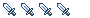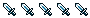## [FND]Dunce Hat

All development of pixel art, maps and other graphics.
kr0n05931
KnightPosts: 652
Joined: 26 Jul 2008, 02:59
Location: United Socialist States of America
Contact:

### [FND]Dunce Hat

A dunce hat to replace those nasty error messages:
Attachmentsdunce-hat.png (665 Bytes) Viewed 804 times
Last edited by kr0n05931 on 16 Dec 2008, 19:33, edited 1 time in total.
Lazy bum.
CrushPosts: 8046
Joined: 25 Aug 2005, 17:08
Location: Germany

### Re: [WIP]Dunce Hat

I think that's a bad idea. When something is wrong the users should notice it so that they report the error to us.

When we hide the error with a placeholder which looks like a legit item people will think that it is just a new item they don't know yet and won't report it.
• former Manasource Programmer
• former TMW Pixel artist
• NOT a game master

Please do not send me any inquiries regarding player accounts on TMW.

You might have heard a certain rumor about me. This rumor is completely false. You might also have heard the other rumor about me. This rumor is 100% accurate.
kr0n05931
KnightPosts: 652
Joined: 26 Jul 2008, 02:59
Location: United Socialist States of America
Contact:

### Re: [WIP]Dunce Hat

Well, we could always use it for another purpose, such as a quest.
Lazy bum.
RotonenPosts: 3154
Joined: 08 Sep 2004, 20:48
Location: Bern, Switzerland

### Re: [WIP]Dunce Hat

I like the error text too. It's hard to get the message wrong with it.
This message used to be meaningful.
kr0n05931
KnightPosts: 652
Joined: 26 Jul 2008, 02:59
Location: United Socialist States of America
Contact:

### Re: [WIP]Dunce Hat

here is the finished .xml file:

Code: Select all

``````<?xml version="1.0"?>
<sprite>
<imageset name="base" src="graphics/sprites/item010.png" width="19" height="19" />

<action name="stand" imageset="base">
<animation direction="down">
<frame index="0" offsetX="-1" offsetY ="-50" />
</animation>
<animation direction="left">
<frame index="1" offsetX="-1" offsetY ="-50"/>
</animation>
<animation direction="up">
<frame index="1" offsetX="-2" offsetY ="-50"/>
</animation>
<animation direction="right">
<frame index="1" offsetX="0" offsetY ="-50"/>
</animation>
</action>

<action name="walk" imageset="base">
<animation direction="down">
<frame index="0" offsetX="-1" offsetY ="-49" delay="75"/>
<frame index="0" offsetX="-1" offsetY ="-50" delay="75" />
<frame index="0" offsetX="-1" offsetY ="-50" delay="75" />
<frame index="0" offsetX="-1" offsetY ="-49" delay="75" />
<frame index="0" offsetX="-1" offsetY ="-50" delay="75" />
<frame index="0" offsetX="-1" offsetY ="-50" delay="75" />
</animation>
<animation direction="left">
<frame index="1" offsetX="-1" offsetY ="-49" delay="75"/>
<frame index="1" offsetX="-1" offsetY ="-48" delay="75"/>
<frame index="1" offsetX="-1" offsetY ="-49" delay="75"/>
<frame index="1" offsetX="-1" offsetY ="-49" delay="75"/>
<frame index="1" offsetX="-1" offsetY ="-48" delay="75"/>
<frame index="1" offsetX="-1" offsetY ="-49" delay="75"/>
</animation>
<animation direction="up">
<frame index="1" offsetX="-2" offsetY ="-50" delay="75"/>
<frame index="1" offsetX="-2" offsetY ="-51" delay="75"/>
<frame index="1" offsetX="-2" offsetY ="-51" delay="75"/>
<frame index="1" offsetX="-2" offsetY ="-50" delay="75"/>
<frame index="1" offsetX="-2" offsetY ="-51" delay="75"/>
<frame index="1" offsetX="-2" offsetY ="-51" delay="75"/>
</animation>
<animation direction="right">
<frame index="1" offsetX="0" offsetY ="-49" delay="75"/>
<frame index="1" offsetX="0" offsetY ="-48" delay="75"/>
<frame index="1" offsetX="0" offsetY ="-49" delay="75"/>
<frame index="1" offsetX="0" offsetY ="-49" delay="75"/>
<frame index="1" offsetX="0" offsetY ="-48" delay="75"/>
<frame index="1" offsetX="0" offsetY ="-49" delay="75"/>
</animation>
</action>

<action name="attack" imageset="base">
<animation direction="down">
<frame index="0" offsetX="-1" offsetY ="-48" delay="75" />
<frame index="0" offsetX="-1" offsetY ="-49" delay="75" />
<frame index="0" offsetX="-1" offsetY ="-47" delay="75" />
<frame index="0" offsetX="-1" offsetY ="-45" delay="75" />
<end />
</animation>
<animation direction="left">
<frame index="1" offsetX="3" offsetY ="-51" delay="75" />
<frame index="1" offsetX="3" offsetY ="-52" delay="75" />
<frame index="1" offsetX="0" offsetY ="-50" delay="75" />
<frame index="1" offsetX="-1" offsetY ="-49" delay="75" />
<end />
</animation>
<animation direction="up">
<frame index="1" offsetX="-3" offsetY ="-50" delay="75"/>
<frame index="1" offsetX="-3" offsetY ="-50" delay="75"/>
<frame index="1" offsetX="-3" offsetY ="-50" delay="75"/>
<frame index="1" offsetX="-3" offsetY ="-49" delay="75"/>
<end />
</animation>
<animation direction="right">
<frame index="1" offsetX="-3" offsetY ="-51" delay="75"/>
<frame index="1" offsetX="-3" offsetY ="-51" delay="75"/>
<frame index="1" offsetX="0" offsetY ="-50" delay="75"/>
<frame index="1" offsetX="1" offsetY ="-49" delay="75"/>
<end />
</animation>
</action>

<action name="attack_bow" imageset="base">
<animation direction="down">
<frame index="0" offsetX="-1" offsetY ="-49" delay="75" />
<frame index="0" offsetX="-1" offsetY ="-49" delay="75" />
<frame index="0" offsetX="-1" offsetY ="-49" delay="75" />
<frame index="0" offsetX="-1" offsetY ="-48" delay="75" />
<frame index="0" offsetX="-1" offsetY ="-49" delay="75" />
<end />
</animation>
<animation direction="left">
<frame index="1" offsetX="2" offsetY ="-50" delay="75" />
<frame index="1" offsetX="2" offsetY ="-50" delay="75" />
<frame index="1" offsetX="2" offsetY ="-50" delay="75" />
<frame index="1" offsetX="4" offsetY ="-51" delay="75" />
<frame index="1" offsetX="4" offsetY ="-51" delay="75" />
<end />
</animation>
<animation direction="up">
<frame index="1" offsetX="-2" offsetY ="-50" delay="75"/>
<frame index="1" offsetX="-2" offsetY ="-50" delay="75"/>
<frame index="1" offsetX="-3" offsetY ="-50" delay="75"/>
<frame index="1" offsetX="-3" offsetY ="-49" delay="75"/>
<frame index="1" offsetX="-3" offsetY ="-50" delay="75"/>
<end />
</animation>
<animation direction="right">
<frame index="1" offsetX="-3" offsetY ="-50" delay="75"/>
<frame index="1" offsetX="-3" offsetY ="-50" delay="75"/>
<frame index="1" offsetX="-3" offsetY ="-50" delay="75"/>
<frame index="1" offsetX="-5" offsetY ="-51" delay="75"/>
<frame index="1" offsetX="-5" offsetY ="-51" delay="75"/>
<end />
</animation>
</action>

<action name="sit" imageset="base">
<animation direction="down">
<frame index="0" offsetX="-1" offsetY ="-38" />
</animation>
<animation direction="left">
<frame index="1" offsetX="3" offsetY ="-40"/>
</animation>
<animation direction="up">
<frame index="1" offsetX="-1" offsetY ="-42"/>
</animation>
<animation direction="right">
<frame index="1" offsetX="-6" offsetY ="-40"/>
</animation>
</action>